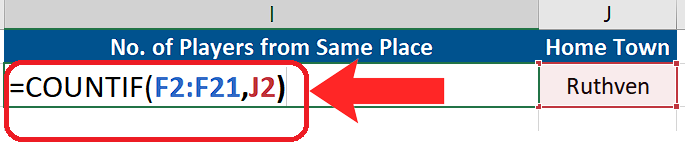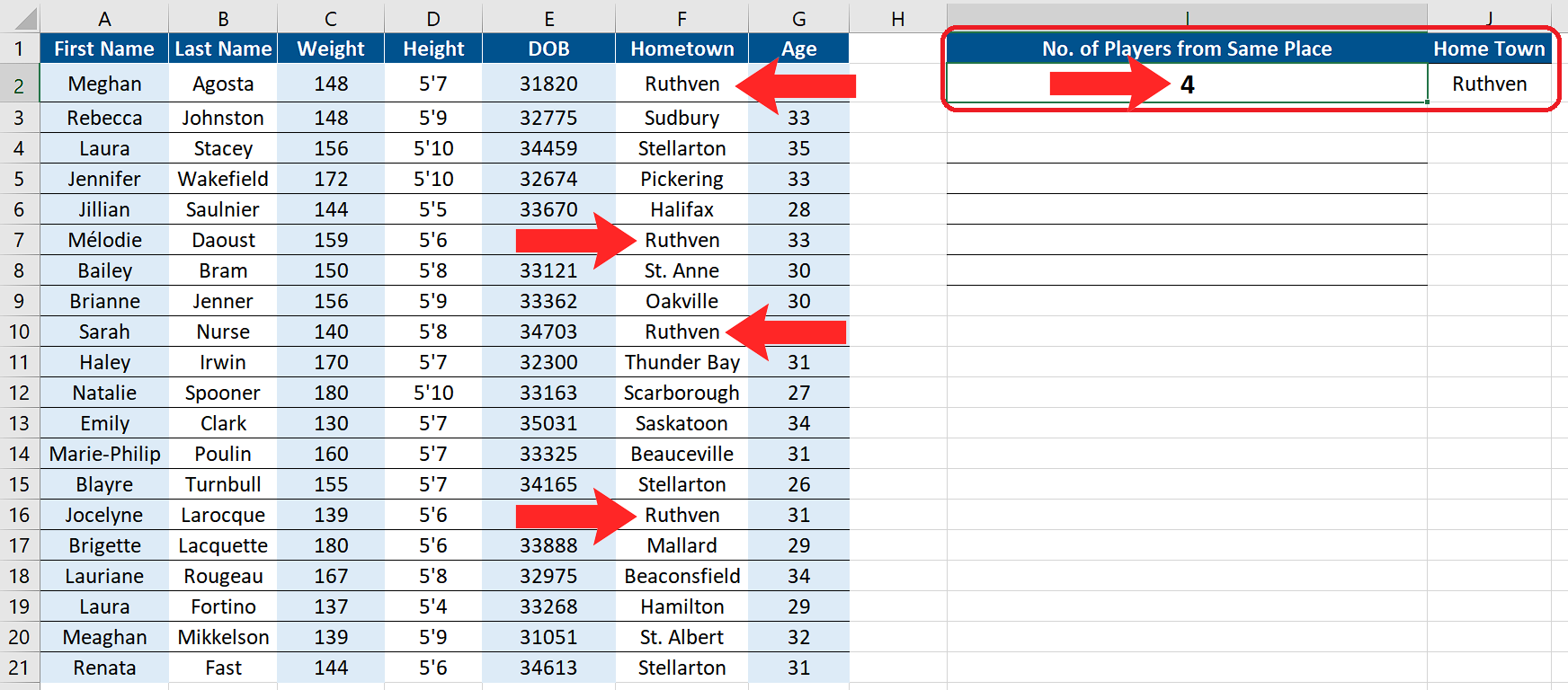# How to count duplicates in Excel

In today’s tutorial we’ll learn how to count the duplicates in any data set. Let’s take an example of the members of a Hockey team and we have some details about each player. Suppose we wish to find out how many players are from the same hometown. We will explore how to achieve this goal by following the steps below.While working with large data sets, it is often required that we find duplicate entries for any reason. Maybe we want to find the duplicates just to count how many times a person’s name has appeared in a data set or we may want to remove the duplicates.

### Step 1 – Create the formula to count duplicates including the first occurrence– We will be using the COUNTIF Function for finding the duplicates in the given data set. However, we’ll have to tailor this function to fulfil our requirements. The custom formula will be as follows. The formula is also shown in the figure.

=COUNTIF(F2:F21, J2)

– Where F2:F21 is the range where we wish to find the duplicates and J2 will hold the value for which we are going to find the duplicates.

### Step 2 – Implement the formula and count duplicates– We will press enter to implement the formula and the result will be displayed in the cell as shown above.

If we wish to exclude the first occurrence and want to only find the number of duplicates then we can subtract 1 from the total number of counts. In that case this will be the formula;

=COUNTIF(Range to find duplicates, Value to find) – 1